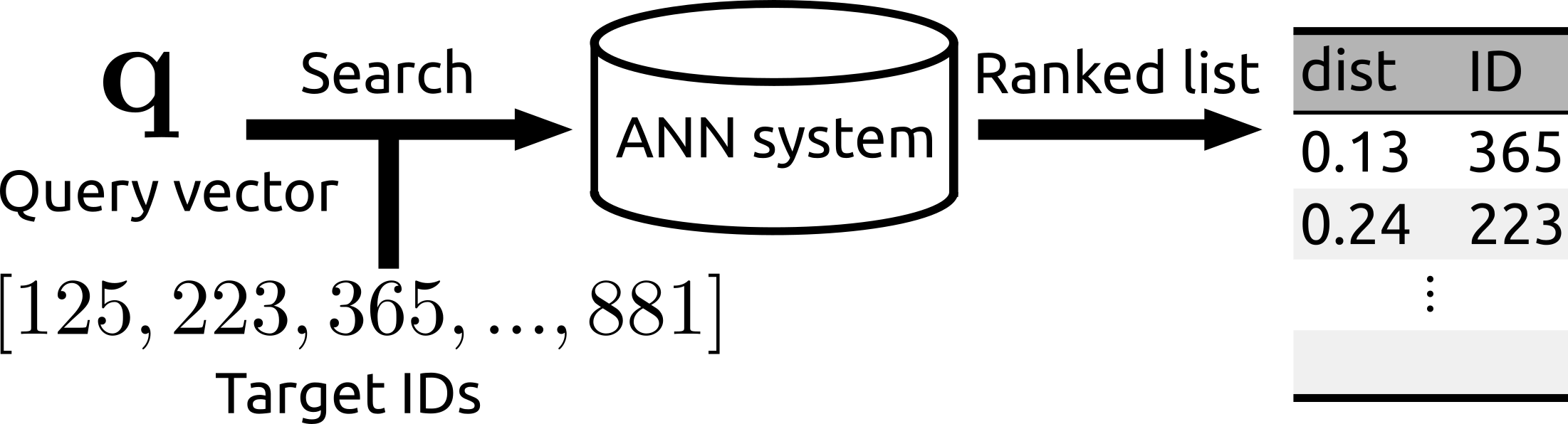# rii

Showing:

0

109

1mo ago

6

## Package

0

MIT

### CategoriesReconfigurable Inverted Index (Rii): IVFPQ-based fast and memory efficient approximate nearest neighbor search method with a subset-search functionality.

Reference:

## Summary of featuresThe search can be operated for a subset of a database.Rii remains fast even after many new items are added.
• Fast and memory efficient ANN. Rii enables you to run billion-scale search in less than 10 ms.
• You can run the search over a subset of the whole database
• Rii Remains fast even after many vectors are newly added (i.e., the data structure can be reconfigured)

## Installing

You can install the package via pip. This library works with Python 3.5+ on linux/mac/wsl/Windows10 (x64, using MSVC:flags - /arch:AVX2, /openmp:llvm, /fp:fast').

``````pip install rii
``````
For windows (maintained by @ashleyabraham)

### Pre-compiled binary for Windows 10 (, may need MS Visual Studio Build tools)

``````pip install https://github.com/ashleyabraham/rii/releases/download/v0.2.7/rii-0.2.7-cp38-cp38-win_amd64.whl
``````

### OpenMP

In order to use OpenMP 3.0 /openmp:llvm flag is used which causes warnings of multiple libs loading, use at your descretion when used with other parallel processing library loadings. To supress use

`SET KMP_DUPLICATE_LIB_OK=TRUE`

### SIMD

The /arch:AVX2 flag is used in MSVC to set appropriate SIMD preprocessors and compiler intrinsics

## Usage

### Basic ANN

``````import rii
import nanopq
import numpy as np

N, Nt, D = 10000, 1000, 128
X = np.random.random((N, D)).astype(np.float32)  # 10,000 128-dim vectors to be searched
Xt = np.random.random((Nt, D)).astype(np.float32)  # 1,000 128-dim vectors for training
q = np.random.random((D,)).astype(np.float32)  # a 128-dim vector

# Prepare a PQ/OPQ codec with M=32 sub spaces
codec = nanopq.PQ(M=32).fit(vecs=Xt)  # Trained using Xt

# Instantiate a Rii class with the codec
e = rii.Rii(fine_quantizer=codec)

# Search
ids, dists = e.query(q=q, topk=3)
print(ids, dists)  # e.g., [7484 8173 1556] [15.06257439 15.38533878 16.16935158]
``````

Note that you can construct a PQ codec and instantiate the Rii class at the same time if you want.

``````e = rii.Rii(fine_quantizer=nanopq.PQ(M=32).fit(vecs=Xt))
``````

Furthermore, you can even write them in one line by chaining a function.

``````e = rii.Rii(fine_quantizer=nanopq.PQ(M=32).fit(vecs=Xt)).add_configure(vecs=X)
``````
``````# The search can be conducted over a subset of the database
target_ids = np.array([85, 132, 236, 551, 694, 728, 992, 1234])  # Specified by IDs
ids, dists = e.query(q=q, topk=3, target_ids=target_ids)
print(ids, dists)  # e.g., [728  85 132] [14.80522156 15.92787838 16.28690338]
``````

``````# Add new vectors
X2 = np.random.random((1000, D)).astype(np.float32)
e.add(vecs=X2)  # Now N is 11000
e.query(q=q)  # Ok. (0.12 msec / query)

# However, if you add quite a lot of vectors, the search might become slower
# because the data structure has been optimized for the initial item size (N=10000)
X3 = np.random.random((1000000, D)).astype(np.float32)
e.add(vecs=X3)  # A lot. Now N is 1011000
e.query(q=q)  # Slower (0.96 msec/query)

# In such case, run the reconfigure function. That updates the data structure
e.reconfigure()
e.query(q=q)  # Ok. (0.21 msec / query)
``````

### I/O by pickling

``````import pickle
with open('rii.pkl', 'wb') as f:
pickle.dump(e, f)
with open('rii.pkl', 'rb') as f:
e_dumped = pickle.load(f)  # e_dumped is identical to e
``````

### Util functions

``````# Print the current parameters
e.print_params()

# Delete all PQ-codes and posting lists. fine_quantizer is kept.
e.clear()

# You can switch the verbose flag
e.verbose = False

# You can merge two Rii instances if they have the same fine_quantizer
e1 = rii.Rii(fine_quantizer=codec)
e2 = rii.Rii(fine_quantizer=codec)
e1.merge(e2)  # Now e1 contains both X1 and X2

``````

## Rate & Review

Great Documentation0
Easy to Use0
Performant0
Highly Customizable0
Bleeding Edge0
Responsive Maintainers0
Poor Documentation0
Hard to Use0
Slow0
Buggy0
Abandoned0
Unwelcoming Community0
100No reviews found
Be the first to rate

## AlternativesNo alternatives found

## TutorialsNo tutorials found